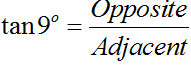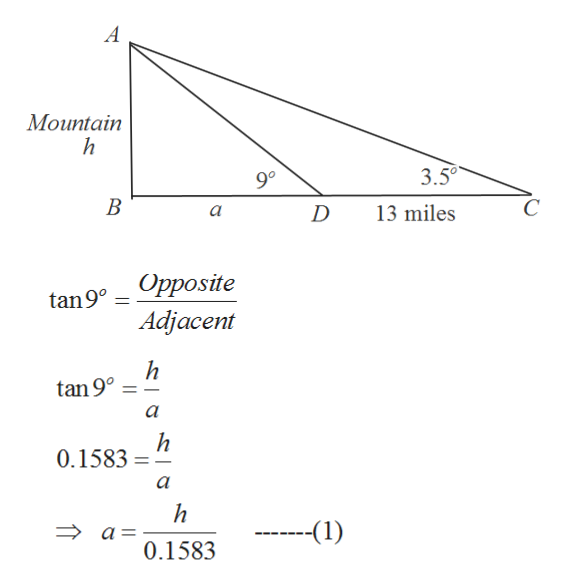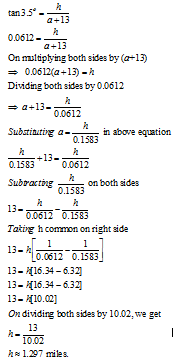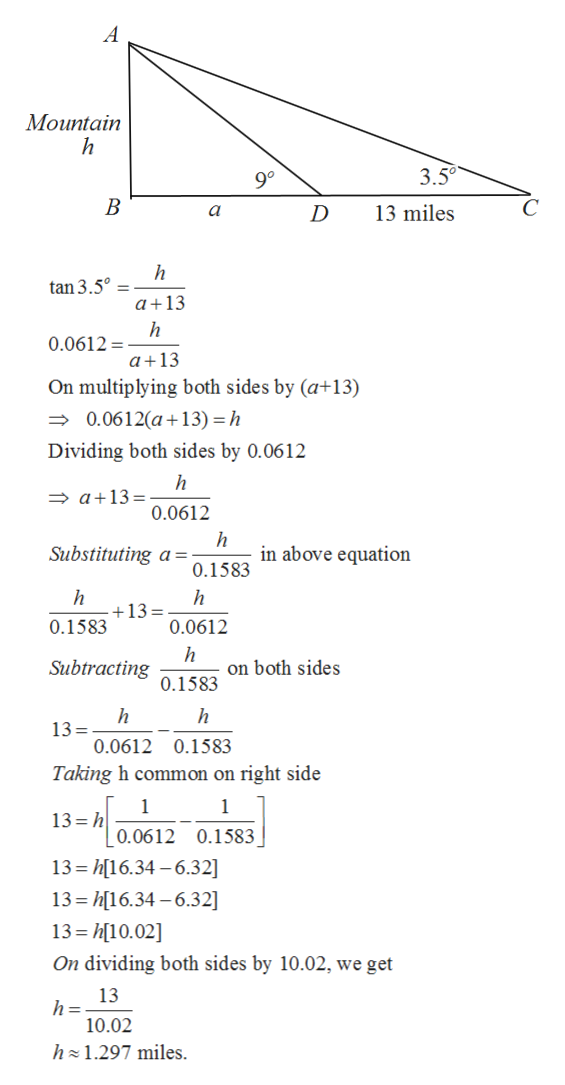# In travelling across flat land, you notice a mountain directly in front of you. Its angle of elevation (to the peak) is 3.5 degrees. After you drive x=13 miles closer to the mountain, the angle of elevation is 9 degrees. Approximate the height of the mountain.

Question
25 views

In travelling across flat land, you notice a mountain directly in front of you. Its angle of elevation (to the peak) is 3.5 degrees. After you drive x=13 miles closer to the mountain, the angle of elevation is 9 degrees. Approximate the height of the mountain.

check_circle

Step 1

First we would draw the diagram of the given situation on the board.

According to figure, AB or h is the height of the mountain. C is my initial position and D is final position.

Step 2

First we will take triangle ABD and will apply tangent ratio.

After applying tangent ratio, we will solve the equaiton for a in terms of h.We got a= h/0.1583.help_outlineImage TranscriptioncloseA Mountain h 3.5 90 13 miles a tan9 Opposite Adjacent tan 9 h 0.1583 a a = -(1) 0.1583 fullscreen
Step 3

Now in triangle ABC, we will apply tangent ratio.

Then we will substitute value of a that is a = h/ 0.1583 and solve for h....help_outlineImage TranscriptioncloseA Mountain 3.5 90 13 miles h tan 3.5° a 13 h 0.0612= a+13 On multiplying both sides by (a+13) 0.0612(a 13) h Dividing both sides by 0.0612 a13 0.0612 h in above equation Substituting 0.1583 h +13 = 0.0612 0.1583 h on both sides Subtracting 0.1583 h 13= 0.0612 0.1583 Taking h common on right side 1 13 h 1 0.0612 0.1583 13 h16.34-6.32] 13 h16.34-6.32] 13 h10.02 On dividing both sides by 10.02, we get 13 h 10.02 h 1.297 miles fullscreen

### Want to see the full answer?

See Solution

#### Want to see this answer and more?

Solutions are written by subject experts who are available 24/7. Questions are typically answered within 1 hour.*

See Solution
*Response times may vary by subject and question.
Tagged in

### Other